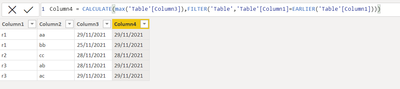cancel
Showing results for
Did you mean:Frequent Visitor

## Calculated Colums with multiple tables

So I have 2 tables and using some formula in the calculated columns section i want to create a new column. It looks like this:

Table 1:

reference | some string | datetime

r1 | aa | 29/11/2021

r1 | bb | 25/11/2021

r2 | cc | 28/11/2021

r3 | ab | 28/11/2021

r3 | ab | 29/11/2021

For table 2 I want to add a calculated column that includes the most recent date for each reference. It would look like this:

Table 2:

reference | calculated max date

r1 | 29/11/2021

r2 | 28/11/2021

r3 | 29/11/2021

I have tried to program it like this:

``````DateSolved =
If (
ServiceTickets[ServiceTicketStateId] = 4,
Calculate(MAX(Mail[DateTimeSent]), Mail[Meldingsnummer] = ServiceTickets[Reference])
)``````

This returns difficulties with the filter expression as it does not allow different tables to be used. How can I solve this? Thanks in advance!

1 ACCEPTED SOLUTIONSuper User

Hi @MagisKoen ,Column4 = CALCULATE(max('Table'[Column3]),FILTER('Table','Table'[Column1]=EARLIER('Table'[Column1])))

result is as below,Please like and mark it as a solution if it give right answer to you. thanks

2 REPLIES 2Super User

Hi @MagisKoen ,Column4 = CALCULATE(max('Table'[Column3]),FILTER('Table','Table'[Column1]=EARLIER('Table'[Column1])))

result is as below,Please like and mark it as a solution if it give right answer to you. thanksFrequent Visitor

Thank you a lot Aburar_123, I did need to put in a Lookupvalue to transpose the data to the other table but this works!